## A voltage source of 10 V is connected to a series RC circuit where R = 2.0 ´ 106 W , and C = 3.0 µF. Find the amount of time required for th

Question

A voltage source of 10 V is connected to a series RC circuit where R = 2.0 ´ 106 W , and C = 3.0 µF. Find the amount of time required for the current in the circuit to decay to 5% of its original value. Hint: This is the same amount of time for the capacitor to reach 95% of its maximum charge.

in progress 0
6 months 2021-08-22T05:28:49+00:00 1 Answers 0 views 0

## Answers ( )

0.5 s

Explanation:

The discharge current in a series RC circuit is given by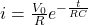where i₀ = V₀/R

For the current i to decay to 5%i₀, i/i₀ = 0.05. Where R = resistance = 2.0 × 10⁶ Ω and C = capacitance = 3.0 μF = 3.0 10⁻⁶ F

So,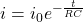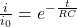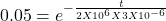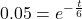taking natural logarithm of both sides

-t /6 = ln(0.05)

t = -ln(0.05)/6 = 0.5 s

So it takes the current 0.5 s to reach 5% of its original value